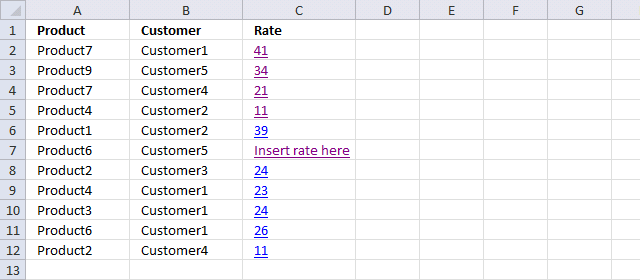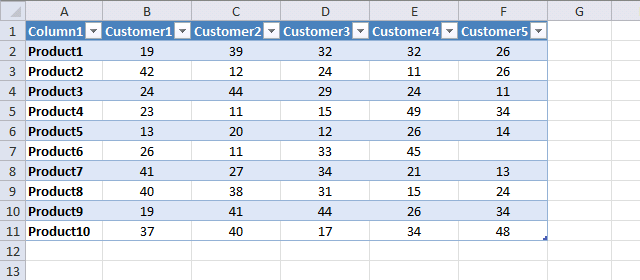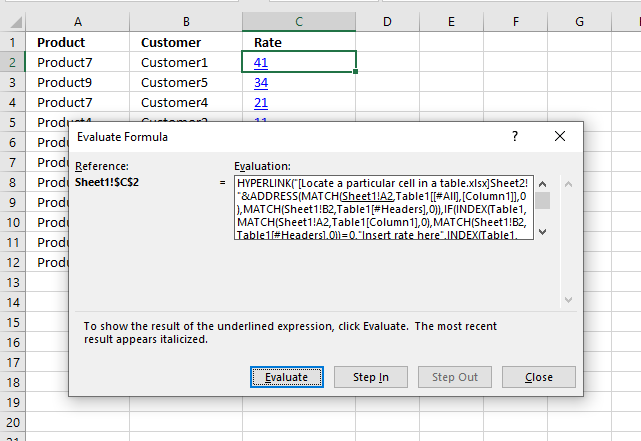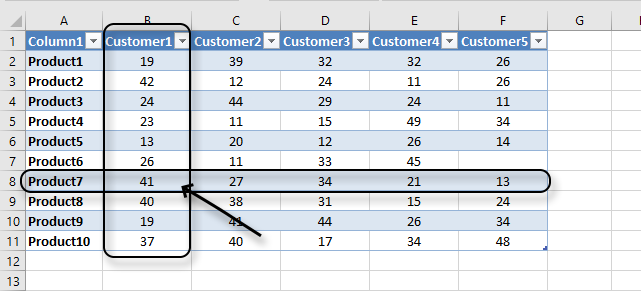Author: Oscar Cronquist Article last updated on March 18, 2020The image above shows a formula in cell C2 that searches for a value based on two conditions specified in column A and B. The image below shows an Excel Table containing values.

The formula returns a value based on a condition that matches a particular header name (horizontal lookup) and another condition that matches a value in the first column (vertical lookup), see image below.

For example, the formula in cell C2 in the image above looks for Product7 in column1 and Customer1 in Table headers and returns the intersecting value, in this case, 41 from the Excel Table. This is called a two-dimensional lookup, read this if you want to learn more: How to perform a two-dimensional lookup

The formula also creates automatically a hyperlink pointing to that particular value in the Excel Table, that is why you see values formatted as hyperlinks in column C.

This makes it really easy to find the value in the Excel Table, simply press with left mouse button on the hyperlink and you will be instantly taken to the correct value in the Excel Table.I have a table with customers as columns & products as rows. Price may different to each customer and product. I am using the following formula to find out the rate of an item for a particular customer;

=IF(INDEX(TABLE3, MATCH(REF_ID, INDEX(TABLE3, , 1), 0), MATCH(REF_prod, INDEX(TABLE3, 1, ), 0))<=0, "PLEASE INSERT A RATE", (INDEX(TABLE3, MATCH(REF_ID, INDEX(TABLE3, , 1), 0), MATCH(REF_prod, INDEX(TABLE3, 1, ), 0))))

Now I want to locate/hyperlink the particular cell intersecting the customer reference and product reference.

The formula in column C searches for the product and the customer in the table below and returns the intersecting value.

Press with mouse on a value in column C and you will instantly select the returned value in the Excel Table.

Product6 / Customer5 does not have a value in the table so the formula tells you to insert a value here, see row 7 above.

Array formula in cell C2, sheet1:

=HYPERLINK("[Locate a particular cell in a table.xlsx]Sheet2!"&ADDRESS(MATCH(Sheet1!A2, Table1[[#All], [Column1]], 0), MATCH(Sheet1!B2, Table1[#Headers], 0)), IF(INDEX(Table1, MATCH(Sheet1!A2, Table1[Column1], 0), MATCH(Sheet1!B2, Table1[#Headers], 0))=0, "Insert rate here", INDEX(Table1, MATCH(Sheet1!A2, Table1[Column1], 0), MATCH(Sheet1!B2, Table1[#Headers], 0))))

You don't need to enter this formula as an array formula yf you are an Excel 365 subscriber, simply press Enter.

#### How to enter an array formula

1. Select cell C2
2. Copy (Ctrl + c) and paste (Ctrl + v) above array formula to Excel
3. Press and hold CTRL + SHIFT simultaneously.
4. Press Enter.
5. Release all keys.

If you did it right, the formula begins and ends with curly brackets, like this:
{=array_formula}

Do not enter these characters yourself, they appear automatically.

### Explaining array formula in cell C2Excel provides a tool that can help you examine, evaluate and troubleshoot a formula, press with left mouse button on the cell that contains the formula you want to check. In this case, we are going to evaluate cell C2.

Cell C2 contains a formula that creates a hyperlink which prevents us from selecting it, if you press with left mouse button on it you will be navigated to another cell in the Excel Table. We need to use the arrow keys to select cell C2 or press with right mouse button on with the mouse to select cell C2.

Go to tab "Formulas" on the ribbon. Press with mouse on "Evaluate Formula" button to open the "Evaluate Formula" dialog box. The "Evaluate" button lets you see the calculations step by step, then press with left mouse button on "Close" button to dismiss the dialog box.

#### Step 1 - Find row number of the matching product value

The MATCH function returns the relative position of an item in an array or cell reference that matches a specified value in a specific order.

MATCH(lookup_value, lookup_array, [match_type])

The third argument in the function is 0 (zero), this means that it will perform an exact match. Table1[Column1] is a structured reference, in other words, a reference to a column named "Column1" in an Excel Table named Table1.

MATCH(Sheet1!A2, Table1[Column1], 0)

becomes

MATCH("Product7",{"Product1"; "Product2"; "Product3"; "Product4"; "Product5"; "Product6"; "Product7"; "Product8"; "Product9"; "Product10"}, 0)

and returns 7. "Poduct7" is the seventh value in the array counting from left to right.

#### Step 2 - Find column number of the matching customer value

This part of the formula calculates a number representing the column in the Excel Table that we want to extract a value from.

becomes

MATCH("Customer1", {"Column1", "Customer1", "Customer2", "Customer3", "Customer4", "Customer5"}, 0)

and returns 2. "Customer1" is the second value in the array.

#### Step 3 - Return intersecting value

The INDEX function returns a value based on the row and column number calculated in the two previous steps.

INDEX(Table1, MATCH(Sheet1!A2, Table1[Column1], 0), MATCH(Sheet1!B2, Table1[#Headers], 0))

becomes

INDEX(Table1, 7, 2)

and returns 41. The image below shows the intersecting value based on the relative row and column number. "Relative" meaning rows and columns in the Excel Table, not in the worksheet.#### Step 4 -  Check if value is 0 (zero) and return  intersecting value or "Insert rate"

The IF function returns text string "Insert rate here" if the formula returns a blank (nothing) and the value if it returns anything else.

IF(logical_test, [value_if_true], [value_if_false])

IF(INDEX(Table1, MATCH(Sheet1!A2, Table1[Column1], 0), MATCH(Sheet1!B2, Table1[#Headers], 0))=0, "Insert rate here", INDEX(Table1, MATCH(Sheet1!A2, Table1[Column1], 0), MATCH(Sheet1!B2, Table1[#Headers], 0)))

becomes

IF(41=0, "Insert rate here", 41)

becomes

IF(FALSE, "Insert rate here", 41)

and returns 41.

#### Step 5 - Calculate address to intersecting value

The ADDRESS function calculates the cell reference needed to build the hyperlink.

"[Locate a particular cell in a table.xlsx]Sheet2!"&ADDRESS(MATCH(Sheet1!A2, Table1[[#All], [Column1]], 0), MATCH(Sheet1!B2, Table1[#Headers], 0))

becomes

"[Locate a particular cell in a table.xlsx]Sheet2!"&ADDRESS(8, 2)

becomes

"[Locate a particular cell in a table.xlsx]Sheet2!"&\$B\$8

and returns [Locate a particular cell in a table.xlsx]Sheet2!\$B\$8

#### Step 6 - Create hyperlink